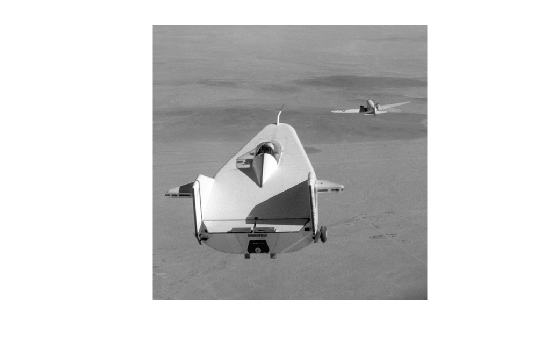getNumberFormatFcn

Get function handle that converts numeric value into character vector

Description

example

fun = getNumberFormatFcn(imgmodel) returns a handle to a function that converts a single numeric value into a character vector for image model imgmodel.

Examples

collapse all

Pixel values obtained from an imagemodel object can be returned in several formats suitable for display in different interactive image processing tools.

Create an image model associated with a grayscale image.

h = imshow('liftingbody.png');im = imagemodel(h)

im =

IMAGEMODEL object accessing an image with these properties:

ClassType: 'uint8'
DisplayRange: [0 255]
ImageHeight: 512
ImageType: 'intensity'
ImageWidth: 512
MinIntensity: 0
MaxIntensity: 255

Select a pixel by specifying the row and column coordinates. This pixel has (row, column) coordinates (50, 250).

r = 50;
c = 250;

Get the numeric value of the pixel using the getPixelValue function.

pxValue = getPixelValue(im,r,c)
pxValue = uint8
151

Convert the numeric pixel value to a string. First, get a function formatFcn that formats numeric pixel values by using the getNumberFormatFcn. Then, specify the numeric value of the pixel as the input argument to formatFcn to get the formatted string.

formatFcn = getNumberFormatFcn(im);
pxValueStr = formatFcn(pxValue)
pxValueStr =
'151'

Get the default pixel information string using the getDefaultPixelInfoString function. This string depends on the type of image but does not use the pixel values. The pixel information string is suitable for use with the Pixel Information tool.

defaultPxInfoStr = getDefaultPixelInfoString(im)
defaultPxInfoStr =
'Intensity'

Using the same string format, get the pixel information string for the specified pixel by using the getPixelInfoString function.

pxInfoStr = getPixelInfoString(im,r,c)
pxInfoStr =
'151'

Get the default pixel region string using the getDefaultPixelRegionString function. This string depends on the type of image but does not use the pixel values. The pixel region string is suitable for use with the Pixel Region tool.

defaultPxRegStr = getDefaultPixelRegionString(im)
defaultPxRegStr =
'000'

There are two steps to get the pixel region string for the specified pixel in the same string format. First, get a function formatFcn that formats numeric pixel values by using the getPixelRegionFormatFcn function. Then, specify the row and column coordinate of the pixel as input arguments to formatFcn to get the formatted string.

formatFcn = getPixelRegionFormatFcn(im);
pxRegStr = formatFcn(r,c)
pxRegStr = 1x1 cell array
{'151'}

Input Arguments

collapse all

Image model, specified as a scalar imagemodel object.

Output Arguments

collapse all

Number format function that converts numeric values into character vectors, returned as a function handle. fun accepts one input argument, a numeric scalar or logical scalar. fun returns the number as a character vector.

Data Types: function_handle